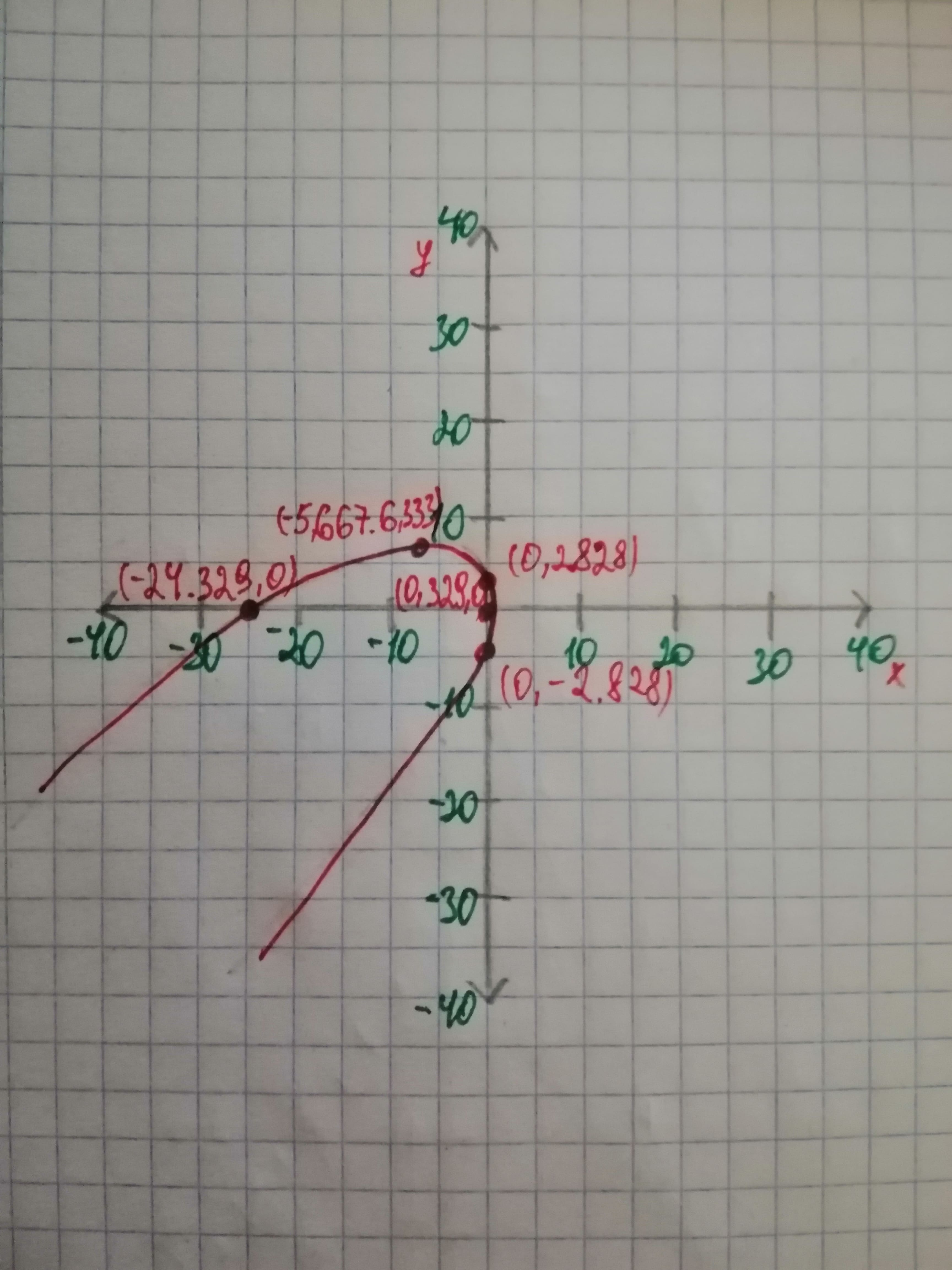Question# The type of the conic section using the Discriminant Test and plot the curve using a computer algebra system.x2 - 2xy + y2 + 24x - 8=0

Conic sections
ANSWEREDThe type of the conic section using the Discriminant Test and plot the curve using a computer algebra system. $$\displaystyle{x}^{2}\ -\ {2}{x}{y}\ +\ {y}^{2}\ +\ {24}{x}\ -\ {8}={0}$$Concept Used: The general equation of conic $$\displaystyle{a}{x}^{2}\ +\ {2}{h}{x}{y}\ +\ {b}{y}^{2}\ +\ {2}{g}{x}\ +\ {2}{f}{y}\ +\ {c}={0}$$ has the discriminant $$\displaystyle\Delta$$ and its given as $$\displaystyle\Delta=\mid{a}{h}{g}{h}{b}{f}{g}{f}{c}\mid={a}{b}{c}\ +\ {2}{f}{g}{h}\ -\ {a}{f}^{2}\ -\ {b}{g}^{2}\ -\ {c}{h}^{2}$$ The general equation of conic $$\displaystyle{a}{x}^{2}\ +\ {2}{h}{x}{y}\ +\ {b}{y}^{2}\ +\ {2}{g}{x}\ +\ {2}{f}{y}\ +\ {c}={0}$$ can also be written in the following way $$\displaystyle{A}{x}^{2}\ +\ {B}{X}{Y}\ +\ {C}{y}^{2}\ +\ {D}{x}\ +\ {E}{y}\ +\ {F}={0}$$ If $$\displaystyle\Delta\ne{q}{0},$$ then the equation is nondegenerate and under this case $$\displaystyle var \neg{{h}}\in{g}\ \text{If}\ {B}^{2}\ -\ {4}{A}{C}\ {>}\ {0},$$ it represents a hyperbola and a rectangular hyperbola if $$\displaystyle{\left({A}\ +\ {C}={0}\right)}$$
$$\displaystyle{v}{a}{r}\neg{h}\in{g}\ \text{If}\ {B}^{2}\ -\ {4}{A}{C}={0},$$ it represents a parabola. $$\displaystyle{v}{a}{r}\neg{h}\in{g}\ \text{If}\ {B}^{2}\ -\ {4}{A}{C}\ {<}\ {0},\text{it represents a circle }{\left({A}={C},\ {B}={0}\right)}$$ or ellipse $$\displaystyle{A}\ne{q}{C}$$ Calculations: The given equation is: $$\displaystyle{x}^{2}\ -\ {2}{x}{y}\ +\ {y}^{2}\ +\ {24}{x}\ -\ {8}={0}$$ and the equation is nondegenerate, hence $$\displaystyle\Delta\ne{q}{0}$$. Now let's find the value of $$\displaystyle{B}^{2}\ -\ {4}{A}{C}$$. From the given equation we have $$\displaystyle{A}={1},\ {B}=\ -{2},\ {C}={1},$$ so on substitution we get $$\displaystyle\Rightarrow\ {B}^{2}\ -\ {4}{A}{C}={\left(-{2}\right)}^{2}\ -\ {4}\ \cdot\ {1}\ \cdot\ {1}\ \Rightarrow\ {B}^{2}\ -\ {4}{A}{C}={4}\ -\ {4}\ \Rightarrow\ {B}^{2}\ -\ {4}{A}{C}={0}$$ Hence the given equation represents a Parabola. The plot of the given curve is as below:Conclusion: The given equation represents a Parabola and the plot is shown above.